# KSEEB Solutions for Class 6 Maths Chapter 8 Decimals Ex 8.6

Students can Download Chapter 8 Decimals Ex 8.6 Questions and Answers, Notes Pdf, KSEEB Solutions for Class 6 Maths helps you to revise the complete Karnataka State Board Syllabus and score more marks in your examinations.

## Karnataka State Syllabus Class 6 Maths Chapter 8 Decimals Ex 8.6

Question 1.
Subtract:-
Solution:
a) Rs 18.25 from Rs 20.75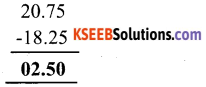b) Rs 202.54 m from Rs 250 m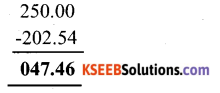c) Rs 5.36 from Rs 8.40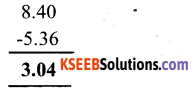d) 2.051 km from 5.20 km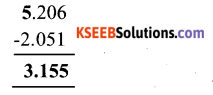e) 0.314 kg from 2.107 kg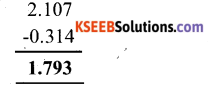Question 2.
Find the value of:-
Solution:
a) 9.756 – 6.28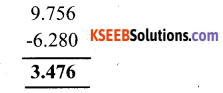b) 21.05 – 15.27c) 18.5 – 6.79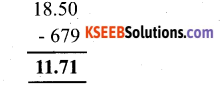d) 11.6 – 9.847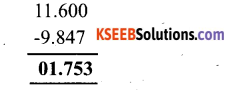Question 3.
Raju bought a book for Rs 35.65. He gave Rs 50 to the shopkeeper. How much money did he get back from the shopkeeper?
Solution:Therefore he will get back Rs. 14.35Question 4.
Rani had Rs 18.50. She bought one ice cream for Rs 11.75. How much money does she have now?
Solution:Question 5.
Tina had 20m 5cm long cloth, she cuts 4 m 50 cm length of cloth from this for making a curtain. How much cloth is left with her?
Solution:
Length of cloth = 20 m 5 cm = 20.05 m
Length of cloth cut so as to make a curtain = 4 m 50 cm = 4.50 m
The length of the left with her will be the difference of these two
Hence, the length of the cloth left with her is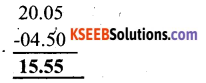15.55 m cloth will be remainingQuestion 6.
Namitha travels 20 km 50 m every day. Out of this she travels 10 km 200m by bus
and the rest by auto. How much distance does she travel by auto?
Solution:
Total distance traveled by namita = 20 km 50m = 20.050 km
Distance traveled by bus = 10 km 200 m = 10.200 km
Distance traveled by auto = Total distance traveled – Distance traveled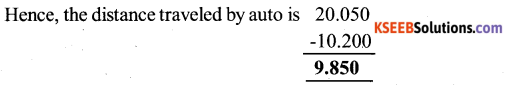Question 7.
Aaksha bought vegetables weighing 10 kg. out of this, 3 kg 500 g is onions, 2 kg 75g is tomatoes and the rest is potatoes. What is the weight of the potatoes?
Solution:
Total Weight of the vegetable = 10.000 kg
Weight of the onions = 3 kg 500 g = 3.500 kg
Weight of the tomatoes = 2 kg 75 g = 2.075 kg
Weight of potatoes = Total weight of vegetables bought
(Weight of onions + weight of tomatoes )
= 10000 – ( 3500 + 2075)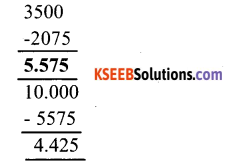Hence, the weight of the potatoes was 4425 kg.